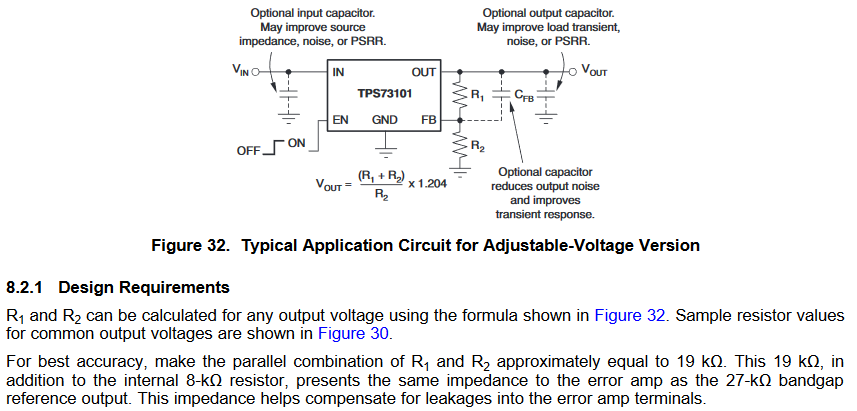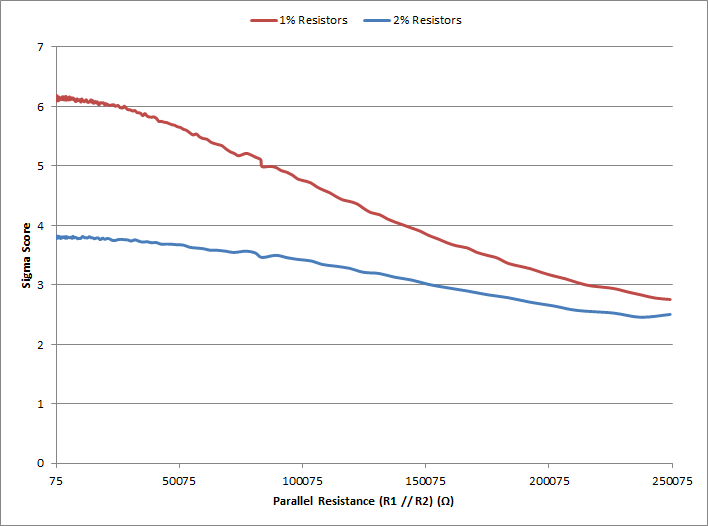If you have a related question, please click the "Ask a related question" button in the top right corner. The newly created question will be automatically linked to this question.

# TPS731: Recommended tolerance for FB resistance

Part Number: TPS731

Hi Team,

Is E24 series usable for FB resistors?

I found that it was necessary to use a 19 kΩ parallel connection for the best output accuracy.

“For best accuracy, make the parallel combination of R1 and R2 approximately equal to 19 kΩ.”

How does the 19kΩ tolerance affect the output?

If the output goes from 19kΩ to the plus direction, is the output also in the plus direction?

Best Regards,

Kenji

• Hi Kenji,

I am working your question and will respond in 1 business day.

Thanks,

- Stephen

• Hello Kenji,

The recommendation on the 19kΩ tolerance is based on limiting the bias current impacts on the setpoint tolerance.
A good description of this is given in the datasheet under section 8.2.1, which I have pasted below.

If you are using the adjustable version of this linear regulator, you can follow the equations in the datasheet to set the output voltage setpoint.
If you lower R2, the output voltage will go up.

Please let me know if you have any further questions.

Thanks,

- Stephen• Hi Stephen-san,

Regards,

Kenji

• Hi Stephen-san,

I understand that R1 || R2 and internal 8kΩ are combined to 27kΩ.

However, what I would like to know more than that is if R1 || R2 has an error with respect to 19kΩ, what is the specific effect?
I'd like an answer such as "how much influence will occur if it shifts by 19% in the + or-direction with respect to 19kΩ?"

I understand that the effect on the actual output is determined by the formula (Vout=(R1+R2)*1.204/R2),
but when that happens, the error of R1 || R2 to 19kΩ will not affect the output. Is that?

What will it affect?
Best Regards,
Kenji
• Hi Kenji-san,

The answer to your question requires a statistical analysis or worst case analysis on a case by case basis.  It depends on the resistors chosen and how much they interact with the bias current.  The acceptable range of resistors also depend on your requirements, such as necessary setpoint tolerance.  To illustrate this I've run a statistical analysis on two sets of designs, and in my analysis I've neglected the benefit the 27kΩ impedance matching will give you, to give you a more generic analysis to apply to other LDO's which do not have this feature.  The analysis run is a 100,000 monte carlo simulation on hundreds of setpoint resistor combinations.  As you can see, there are plenty of design options available if you need a +/- 3 sigma design or better.

To answer the question for your specific application, take the design equation in the datasheet and run a monte carlo analysis in your tool of choice (MS excel, matlab, mathcad, etc).  Please see TI Application Report "AN-1378 Method for Calculating Output Voltage Tolerances in Adjustable Regulators" for more details on statistical analysis.

Hypothetical Requirements

Vref = 1.2V, 1% tolerance
Ibias = 150nA nominal, 99% tolerance (to give a worst case simulation)
Vout_desired = 5V, 3% tolerance for the setpoint
Resistor range 100Ω to 1Meg Ω

E96, 1% series resistors were used for R1 and R2 for the first simulation.
E48, 2% series resistors were used for R1 and R2 for the second simulation.Thanks,

- Stephen

• Hi Stephen,

・ What does SigmaScore mean if it is large or small?

・ I would like to know why R1 // R2 = 19kΩ is optimal from this graph.

Best Regards,

Kenji

• Hi Kenji,

Sigma score is just a term to describe the statistical quality of the design.

If the data set is a gaussian distribution, and your sigma (standard deviation) is +/- 6, then the statistical quality of your design will yield 3.4ppm failures.  So 3.4 units out of a million will statistically measure beyond specification, if your requirements were +/- 6 sigma.

When R1//R2 = 19kΩ, then by design you are minimizing the error due to the adjust current.  The graph attempts to give you other options where you cannot choose R1//R2 = 19kΩ, and you still need a high quality design.

Thanks,

- Stephen